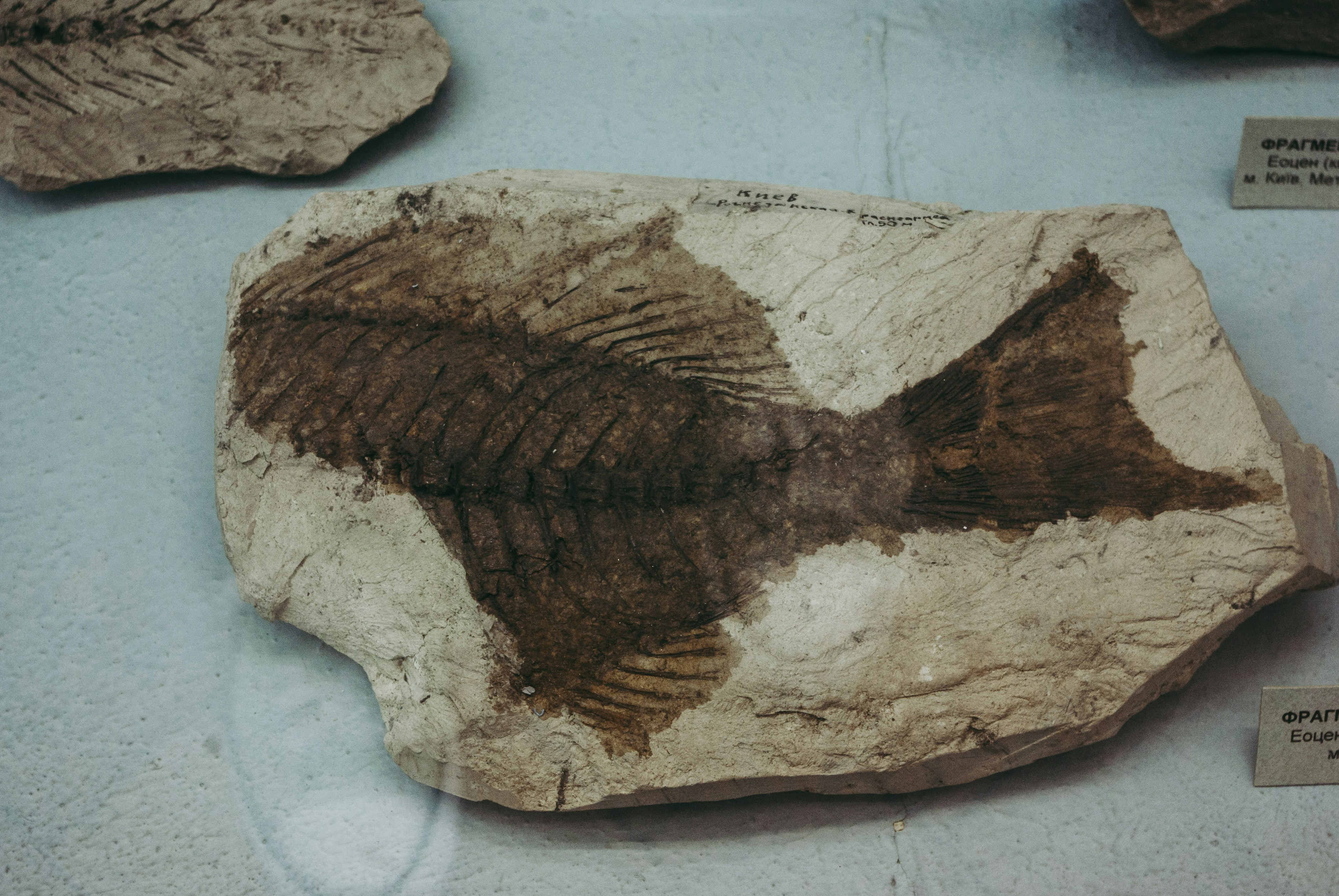Recently PublishedJournal article

## List Graphs and Distance-consistent Node Labelings

Most ViewedJournal article

## The Eccentric-distance Sum of Some Graphs

Let $G = (V,E)$ be a simple connected graph. Theeccentric-distance sum of $G$ is defined as$\xi^{ds}(G) =\ds\sum_{\{u,v\}\subseteq V(G)} [e(u)+e(v)] d(u,v)$, where $e(u)$ %\dsis the eccentricity of the vertex $u$ in $G$ and $d(u,v)$ is thedistance between $u$ and $v$. In this paper, we establish formulaeto calculate the eccentric-distance sum for some graphs, namelywheel, star, broom, lollipop, double star, friendship, multi-stargraph and the join of $P_{n-2}$ and $P_2$.Journal article

## Inverse Graphs Associated with Finite Groups

Let $(\Gamma,*)$ be a finite group and $S$ a possibly empty subset of $\Gamma$ containing its non-self-invertible elements. In this paper, we introduce the inverse graph associated with $\Gamma$ whose set of vertices coincides with $\Gamma$ such that two distinct vertices $u$ and $v$ are adjacent if and only if either $u * v\in S$ or $v * u\in S$. We then investigate its algebraic and combinatorial structures.
Suggested For YouJournal article

## On Some Covering Graphs of a Graph

For a graph $G$ with vertex set $V(G)=\{v_1, v_2, \dots, v_n\}$, let $S$ be the covering set of $G$ having the maximum degree over all the minimum covering sets of $G$. Let $N_S[v]=\{u\in S : uv \in E(G) \}\cup \{v\}$ be the closed neighbourhood of the vertex $v$ with respect to $S.$ We define a square matrix $A_S(G)= (a_{ij}),$ by $a_{ij}=1,$ if $\left |N_S[v_i]\cap N_S[v_j] \right| \geq 1, i\neq j$ and 0, otherwise. The graph $G^S$ associated with the matrix $A_S(G)$ is called the maximum degree minimum covering graph (MDMC-graph) of the graph $G$. In this paper, we give conditions for the graph $G^S$ to be bipartite and Hamiltonian. Also we obtain a bound for the number of edges of the graph $G^S$ in terms of the structure of $G$. Further we obtain an upper bound for covering number (independence number) of $G^S$ in terms of the covering number (independence number) of $G$.
Journal article

## Self-dual Embeddings of K_{4m,4n} in Different Orientable and Nonorientable Pseudosurfaces with the Same Euler Characteristic